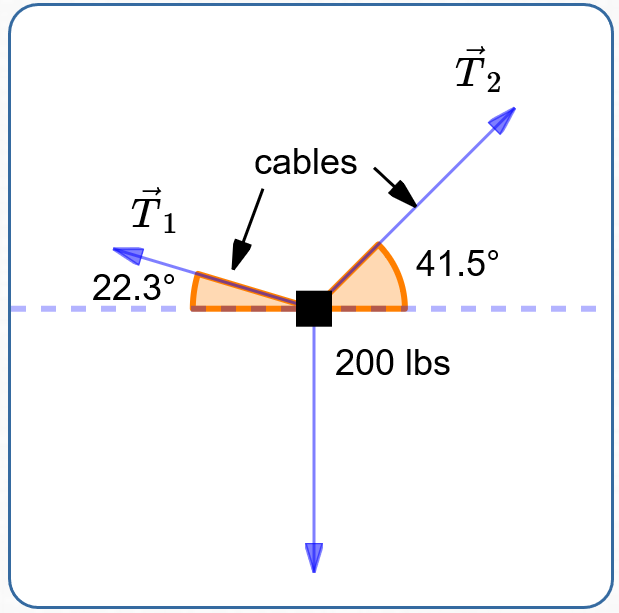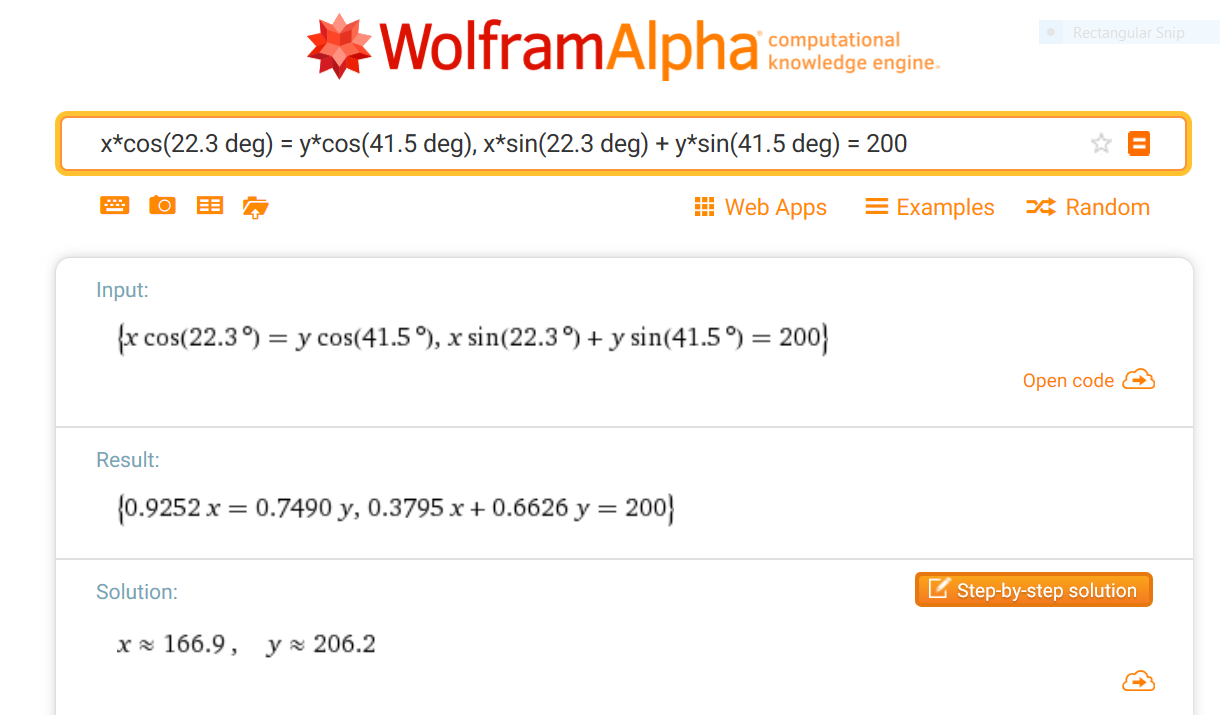﻿ Vector Application: Forces Acting on an Object in Equilibrium

# Vector Application: Forces Acting on an Object in Equilibrium

• PRACTICE (online exercises and printable worksheets)
• You may want to review some prior sections:

Forces are vectors.
Why? Forces have both size and direction.

For example, if you're pushing an object:

• the size of the force is how hard you're pushing
• the direction of the force is the direction that you're pushing

Depending on the definition being used, the weight of an object can be considered as either a vector or a scalar:

• weight as a vector: weight is the force of gravity acting on an object
• weight as a scalar: weight is the size of the force of gravity acting on an object
In the United States, a common unit of weight is the pound, abbreviated as ‘lb’.

 A free-body diagram shows all the forces acting on an object. A typical free-body diagram is shown at right. By definition, an object is in equilibrium if the sum of the forces acting on the object is the zero vector. The zero vector is denoted by ‘$\,\vec 0\,$’. If an object is at rest (not moving), then it is in equilibrium. Note about the other direction: If an object is in equilibrium, then its velocity is not changing—it is either at rest, or moving at a constant velocity.a typical free-body diagram

The notation $\,\|\vec F\|\,$ for the length of a vector $\,\vec F\,$ can get tedious.
Sometimes, a simpler notation is used: let $\,F\,$ (without an arrow) denote the length of $\,\vec F\,$.
This simpler notation will be used in this section.

## Example: Finding Tensions in Cables

 Problem: Two cables are supporting a 200 pound object, as shown at right. Find the tensions in the cables (as vectors). Solution: Let $\,\vec T_1\,$ and $\,\vec T_2\,$ denote the cable tensions, as shown. Let $\,T_1\,$ and $\,T_2\,$ denote the lengths of $\,\vec T_1\,$ and $\,\vec T_2\,$, respectively. The suspended object causes a 200 lb force pointing straight down.Since the object is at rest (assume the cables don't break), it is in equilibrium.
There are different ways to write down the equilibrium condition and then solve for the desired tensions.
Take a look at the variations shown below, and decide which you like best!
The author (Dr. Burns) prefers approach #1.

The methods shown in the table below will use some/all of the following information:

• Size is a nonnegative quantity: i.e., size (length) is always greater than or equal to zero.
• Since $\,T_1\,$ and $\,T_2\,$ (no arrows) are lengths, they are nonnegative.
• For acute angles $\,\theta\,$, $\,\cos\theta\,$ and $\,\sin\theta\,$ are both positive.
• SIZES of components:
 vector size of horizontal component size of vertical component $\,\vec T_1\,$ $T_1\cos 22.3^\circ$ $T_1\sin 22.3^\circ$ $\,\vec T_2\,$ $T_2\cos 41.5^\circ$ $T_2\sin 41.5^\circ$ weight vector 0 200
• SIGNS (plus/minus) of components:
 vector sign of horizontal component sign of vertical component $\,\vec T_1\,$ negative (points to the left) positive (points up) $\,\vec T_2\,$ positive (points to the right) positive (points up) weight vector (0 has no sign) negative (points down)
• ‘Reference angle’ analytic forms of tension vectors:
Recall that the reference angle is the smallest angle between the vector and the $x$-axis.
Just put together the sizes and signs of the components:
• $\vec T_1 = \langle -T_1\cos 22.3^\circ\,,\,T_1\sin 22.3^\circ \rangle$
• $\vec T_2 = \langle T_2\cos 41.5^\circ\,,\,T_2\sin 41.5^\circ \rangle$
• ‘Standard direction’ analytic forms of tension vectors:
• standard direction for $\,\vec T_1\,$ is $\,180^\circ - 22.3^\circ = 157.7^\circ$
• $\vec T_1 = \langle T_1\cos 157.7^\circ\,,\,T_1\sin 157.7^\circ \rangle$
• $\vec T_2 = \langle T_2\cos 41.5^\circ\,,\,T_2\sin 41.5^\circ \rangle$
• Analytic form of Weight Vector:
$\text{weight vector} = \langle 0,-200\rangle$

## Slightly Different Approaches to Satisfy the Equilibrium Condition

Approach #1 is shortest, and is preferred by Dr. Burns.

## Approach #1

• The size of the components pointing left must equal the size of the components pointing right: $$T_1\cos 22.3^\circ = T_2\cos 41.5^\circ$$
• The sum of the sizes of the components pointing up must equal the size of the component pointing down: $$T_1\sin 22.3^\circ + T_2\sin 41.5^\circ = 200$$
SYSTEM OF EQUATIONS TO BE SOLVED: $$\begin{gather} T_1\cos 22.3^\circ = T_2\cos 41.5^\circ\cr T_1\sin 22.3^\circ + T_2\sin 41.5^\circ = 200 \end{gather}$$

Approaches #2 and #3 both use the same idea: for an object in equilibrium, the sum of the forces is the zero vector.
The only difference is the naming of the forces:

• Approach #2 uses the ‘reference angle’ analytic form.
• Approach #3 uses the ‘standard direction’ analytic form.

## Approach #2

• Sum the ‘reference angle’ versions of the forces, and set equal to the zero vector: $$\begin{gather} \vec T_1 + \vec T_2 + \text{weight vector} = \vec 0\cr\cr \langle\ \color{red}{-T_1\cos 22.3^\circ}\,,\,\color{green}{T_1\sin 22.3^\circ} \ \rangle + \langle\ \color{red}{T_2\cos 41.5^\circ}\,,\,\color{green}{T_2\sin 41.5^\circ} \ \rangle + \langle \color{red}{0},\color{green}{-200}\rangle \ =\ \langle \color{red}{0},\color{green}{0}\rangle\cr\cr \langle\ \color{red}{-T_1\cos 22.3^\circ + T_2\cos 41.5^\circ}\ ,\ \color{green}{T_1\sin 22.3^\circ + T_2\sin 41.5^\circ -200}\ \rangle \ =\ \langle \color{red}{0},\color{green}{0}\rangle \end{gather}$$
• For two vectors to be equal, their components must be equal.
SYSTEM OF EQUATIONS TO BE SOLVED: $$\begin{gather} \color{red}{-T_1\cos 22.3^\circ + T_2\cos 41.5^\circ} = \color{red}{0}\cr \color{green}{T_1\sin 22.3^\circ + T_2\sin 41.5^\circ -200} = \color{green}{0} \end{gather}$$

## Approach #3

• Sum the ‘standard direction’ versions of the forces, and set equal to the zero vector: $$\begin{gather} \vec T_1 + \vec T_2 + \text{weight vector} = \vec 0\cr\cr \langle\ T_1\cos 157.7^\circ\,,\,T_1\sin 157.7^\circ \ \rangle + \langle\ T_2\cos 41.5^\circ\,,\,T_2\sin 41.5^\circ \ \rangle + \langle 0,-200\rangle \ =\ \langle 0,0\rangle\cr\cr \langle\ T_1\cos 157.7^\circ + T_2\cos 41.5^\circ\ ,\ T_1\sin 157.7^\circ + T_2\sin 41.5^\circ -200\ \rangle \ =\ \langle 0,0\rangle \end{gather}$$
• For two vectors to be equal, their components must be equal.
SYSTEM OF EQUATIONS TO BE SOLVED: $$\begin{gather} T_1\cos 157.7^\circ + T_2\cos 41.5^\circ = 0\cr T_1\sin 157.7^\circ + T_2\sin 41.5^\circ -200 = 0 \end{gather}$$

## Solve the Resulting System of Equations in $\,T_1\,$ and $\,T_2\,$

Since $\,\cos 157.7^\circ = -\cos 22.3^\circ\,$, a moment's inspection shows that the three systems of equations are equivalent.
Use your favorite technique (perhaps substitution or elimination) to solve the system of your choice.

For your convenience, the system in Approach #1 is solved here:

• From the first equation:
$\displaystyle T_1 = T_2\,\frac{\cos 41.5^\circ}{\cos 22.3^\circ}$
• Substituting into the second equation:
$\displaystyle T_2\,\frac{\cos 41.5^\circ}{\cos 22.3^\circ}(\sin 22.3^\circ) + T_2\sin 41.5^\circ = 200$
• Solving for $\,T_2\,$:
$\displaystyle T_2 = \frac{200}{\frac{\cos 41.5^\circ}{\cos 22.3^\circ}(\sin 22.3^\circ) + \sin 41.5^\circ} \approx 206.230$
• Solving for $\,T_1\,$:
$\displaystyle T_1 = T_2\frac{\cos 41.5^\circ}{\cos 22.3^\circ} \approx 166.943$
• It is most reliable to zip up to WolframAlpha and type in:

x*cos(22.3 deg) = y*cos(41.5 deg), x*sin(22.3 deg) + y*sin(41.5 deg) = 200

Here's a screen shot of the input and result from WolframAlpha:## Find the Tensions in the Cables (as vectors)

• $\vec T_1 = \langle -T_1\cos 22.3^\circ, T_1\sin 22.3^\circ \rangle \approx \langle -154.457 , 63.348 \rangle$
• $\vec T_2 = \langle T_2\cos 41.5^\circ, T_2\sin 41.5^\circ \rangle \approx \langle 154.457 , 136.652 \rangle$

• the horizontal components cancel out: $\,-154.457 + 154.457 = 0$
• the sum of the vertical components is the weight: $\,63.348 + 136.652 = 200$

Master the ideas from this section